Synchrotron radiation (i.e., radiation emitted by a charged particle constrained to follow a circular orbit by a magnetic field) is of particular importance in astrophysics, since much of the observed radio frequency emission from supernova remnants and active galactic nuclei is thought to be of this type.

Consider a charged particle moving in a circle of radiuswith constant angular velocity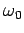. Suppose that the orbit lies in the-plane. The radius vector pointing from the centre of the orbit to the retarded position of the charge is defined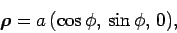(1667)

where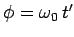is the angle subtended between this vector and the-axis. The retarded velocity and acceleration of the charge take the form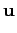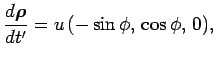(1668)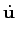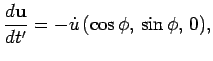(1669)

where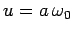and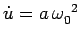. The observation point is chosen such that the radius vector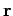, pointing from the retarded position of the charge to the observation point, is parallel to the-plane. Thus, we can write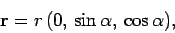(1670)

where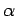is the angle subtended between this vector and the-axis. As usual, we defineas the angle subtended between the retarded radius vectorand the retarded direction of motion of the charge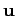. It follows that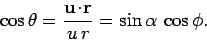(1671)

It is easily seen that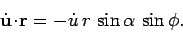(1672)

A little vector algebra shows that(1673)

giving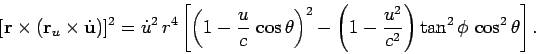(1674)

Making use of Eq. (1659), we obtain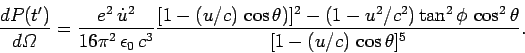(1675)

It is convenient to write this result in terms of the anglesand, instead ofand. After a little algebra we obtain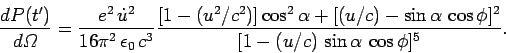(1676)

Let us consider the radiation pattern emitted in the plane of the orbit: i.e.,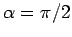, with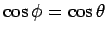. It is easily seen that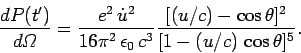(1677)

In the non-relativistic limit, the radiation pattern has a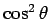dependence. Thus, the pattern is like that of dipole radiation where the axis is aligned along the instantaneous direction of acceleration. As the charge becomes more relativistic, the radiation lobe in the forward direction (i.e.,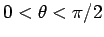) becomes more more focused and more intense. Likewise, the radiation lobe in the backward direction (i.e.,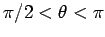) becomes more diffuse. The radiation pattern has zero intensity at the angles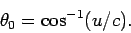(1678)

These angles demark the boundaries between the two radiation lobes. In the non-relativistic limit,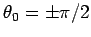, so the two lobes are of equal angular extents. In the highly relativistic limit,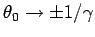, so the forward lobe becomes highly concentrated about the forward direction (). In the latter limit, Eq. (1677) reduces to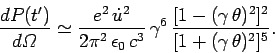(1679)

Thus, the radiation emitted by a highly relativistic charge is focused into an intense beam, of angular extent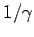, pointing in the instantaneous direction of motion. The maximum intensity of the beam scales like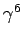.

Integration of Eq. (1676) over all solid angle (using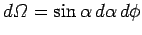) yields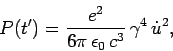(1680)

which agrees with Eq. (1649), provided that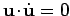. This expression can also be written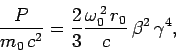(1681)

where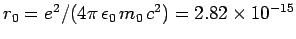meters is the classical electron radius,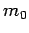is the rest mass of the charge, and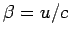. If the circular motion takes place in an orbit of radius, perpendicular to a magnetic field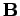, thensatisfies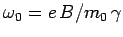. Thus, the radiated power is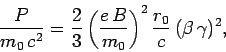(1682)

and the radiated energy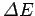per revolution is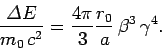(1683)

Let us consider the frequency distribution of the emitted radiation in the highly relativistic limit. Suppose, for the sake of simplicity, that the observation point lies in the plane of the orbit (i.e.,). Since the radiation emitted by the charge is beamed very strongly in the charge's instantaneous direction of motion, a fixed observer is only going to see radiation (at some later time) when this direction points almost directly towards the point of observation. This occurs once every rotation period, when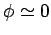, assuming that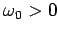. Note that the point of observation is located many orbit radii away from the centre of the orbit along the positive-axis. Thus, our observer sees short periodic pulses of radiation from the charge. The repetition frequency of the pulses (in radians per second) is. Let us calculate the duration of each pulse. Since the radiation emitted by the charge is focused into a narrow beam of angular extent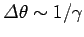, our observer only sees radiation from the charge when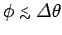. Thus, the observed pulse is emitted during a time interval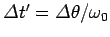. However, the pulse is received in a somewhat shorter time interval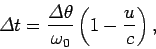(1684)

because the charge is slightly closer to the point of observation at the end of the pulse than at the beginning. The above equation reduces to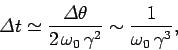(1685)

since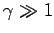and. The width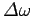of the pulse in frequency space obeys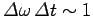. Hence,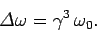(1686)

In other words, the emitted frequency spectrum contains harmonics up to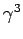times that of the cyclotron frequency,.